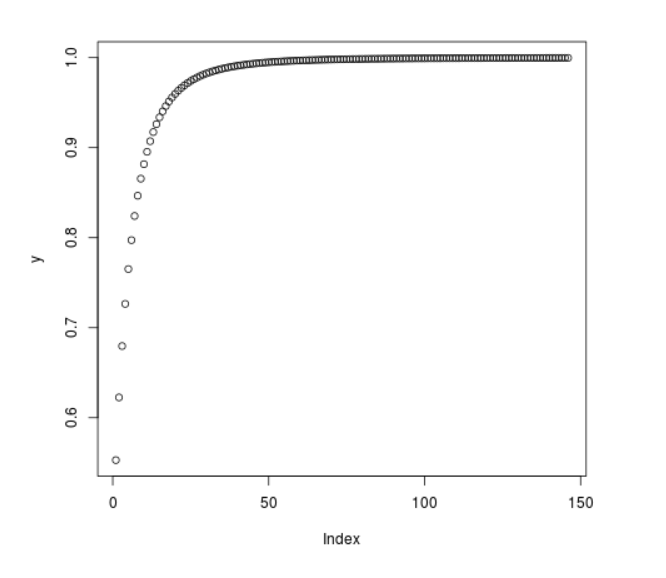Related Articles
Compute the value of F Cumulative Distribution Function in R Programming – pf() Function
• Last Updated : 25 Jun, 2020

`pf()` function in R Language is used to compute the density of F Cumulative Distribution Function over a sequence of numeric values. It also plots a density graph for F Cumulative Distribution.

Syntax: pf(x, df1, df2)

Parameters:
x: Numeric Vector
df: Degree of Freedom

Example 1:

 `# R Program to compute ``# Cumulative F Density`` ` `# Creating a sequence of x-values``x <``-` `seq(``1``, ``30``, by ``=` `2``)`` ` `# Calling pf() Function``y <``-` `pf(x, df1 ``=` `2``, df2 ``=` `3``)``y`

Output:

```  0.5352420 0.8075499 0.8891420 0.9258675 0.9460051 0.9584308 0.9667275
 0.9725899 0.9769124 0.9802073 0.9827867 0.9848509 0.9865331 0.9879255
 0.9890935
```

Example 2:

 `# R Program to compute ``# Cumulative F Density`` ` `# Creating a sequence of x-values``x <``-` `seq(``1``, ``30``, by ``=` `0.2``)`` ` `# Calling pf() Function``y <``-` `pf(x, df1 ``=` `3``, df2 ``=` `7``)`` ` `# Plot a graph``plot(y)`

Output:My Personal Notes arrow_drop_up